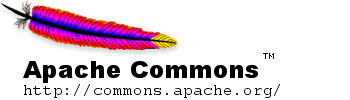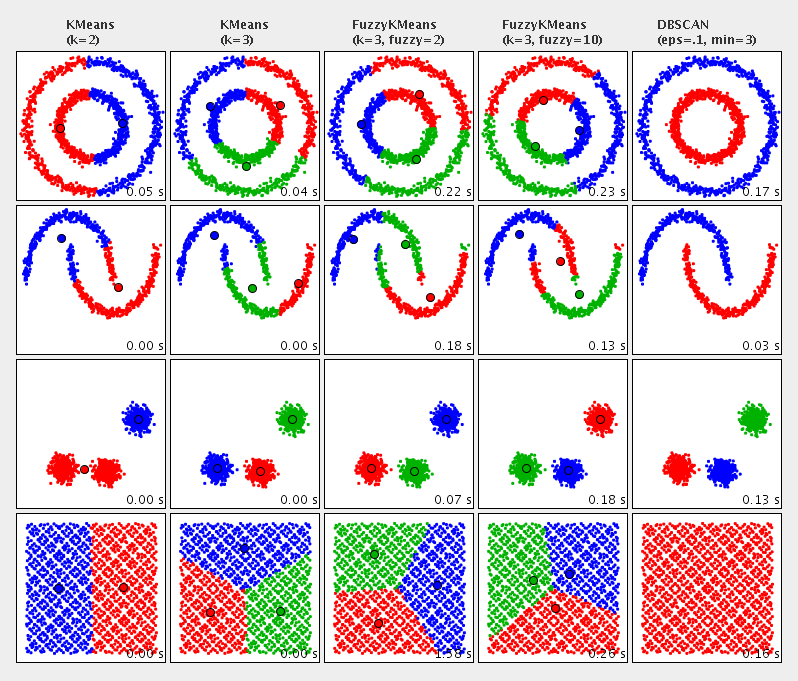## 16 Machine Learning

### 16.1 Overview

Machine learning support in commons-math currently provides operations to cluster data sets based on a distance measure.

### 16.2 Clustering algorithms and distance measures

The Clusterer class represents a clustering algorithm. The following algorithms are available:

• KMeans++: It is based on the well-known kMeans algorithm, but uses a different method for choosing the initial values (or "seeds") and thus avoids cases where KMeans sometimes results in poor clusterings. KMeans/KMeans++ clustering aims to partition n observations into k clusters in such that each point belongs to the cluster with the nearest center.
• Fuzzy-KMeans: A variation of the classical K-Means algorithm, with the major difference that a single data point is not uniquely assigned to a single cluster. Instead, each point i has a set of weights uij which indicate the degree of membership to the cluster j. The fuzzy variant does not require initial values for the cluster centers and is thus more robust, although slower than the original kMeans algorithm.
• DBSCAN: Density-based spatial clustering of applications with noise (DBSCAN) finds a number of clusters starting from the estimated density distribution of corresponding nodes. The main advantages over KMeans/KMeans++ are that DBSCAN does not require the specification of an initial number of clusters and can find arbitrarily shaped clusters.
• Multi-KMeans++: Multi-KMeans++ is a meta algorithm that basically performs n runs using KMeans++ and then chooses the best clustering (i.e., the one with the lowest distance variance over all clusters) from those runs.

An comparison of the available clustering algorithms:### 16.3 Distance measures

Each clustering algorithm requires a distance measure to determine the distance between two points (either data points or cluster centers). The following distance measures are available:

### 16.3 Example

Here is an example of a clustering execution. Let us assume we have a set of locations from our domain model, where each location has a method double getX() and double getY() representing their current coordinates in a 2-dimensional space. We want to cluster the locations into 10 different clusters based on their euclidean distance.

The cluster algorithms expect a list of Clusterable as input. Typically, we don't want to pollute our domain objects with interfaces from helper APIs. Hence, we first create a wrapper object:

// wrapper class
public static class LocationWrapper implements Clusterable {
private double[] points;
private Location location;

public LocationWrapper(Location location) {
this.location = location;
this.points = new double[] { location.getX(), location.getY() }
}

public Location getLocation() {
return location;
}

public double[] getPoint() {
return points;
}
}

Now we will create a list of these wrapper objects (one for each location), which serves as input to our clustering algorithm.
// we have a list of our locations we want to cluster. create a
List<Location> locations = ...;
List<LocationWrapper> clusterInput = new ArrayList<LocationWrapper>(locations.size());
for (Location location : locations)

Finally, we can apply our clustering algorithm and output the found clusters.

// initialize a new clustering algorithm.
// we use KMeans++ with 10 clusters and 10000 iterations maximum.
// we did not specify a distance measure; the default (euclidean distance) is used.
KMeansPlusPlusClusterer<LocationWrapper> clusterer = new KMeansPlusPlusClusterer<LocationWrapper>(10, 10000);
List<CentroidCluster<LocationWrapper>> clusterResults = clusterer.cluster(clusterInput);

// output the clusters
for (int i=0; i<clusterResults.size(); i++) {
System.out.println("Cluster " + i);
for (LocationWrapper locationWrapper : clusterResults.get(i).getPoints())
System.out.println(locationWrapper.getLocation());
System.out.println();
}